2021 NFL MVP Odds
+550
5.5 to 1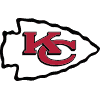Patrick Mahomes
10.1% implied probability

+800
8 to 1Matthew Stafford
7.3% implied probability

+1000
10 to 1Tom Brady
6.0% implied probability

+1000
10 to 1Kyler Murray
6.0% implied probability

+1000
10 to 1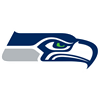Russell Wilson
6.0% implied probability

+1400
14 to 1Dak Prescott
4.4% implied probability

+1600
16 to 1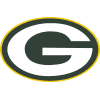Aaron Rodgers
3.9% implied probability

+2000
20 to 1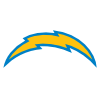Justin Herbert
3.1% implied probability

+2500
25 to 1Jameis Winston
2.5% implied probability

+3500
35 to 1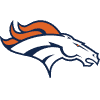Teddy Bridgewater
1.8% implied probability

+3500
35 to 1Jalen Hurts
1.8% implied probability

+3500
35 to 1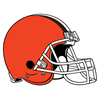Baker Mayfield
1.8% implied probability

+3500
35 to 1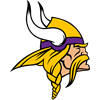Kirk Cousins
1.8% implied probability

+4000
40 to 1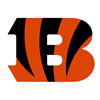Joe Burrow
1.6% implied probability

+4000
40 to 1Christian McCaffrey
1.6% implied probability

+5000
50 to 1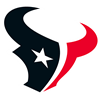Deshaun Watson
1.3% implied probability

+6500
65 to 1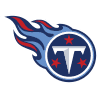Derrick Henry
1.0% implied probability

+6500
65 to 1Sam Darnold
1.0% implied probability

+6500
65 to 1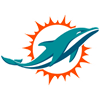Tua Tagovailoa
1.0% implied probability

+6500
65 to 1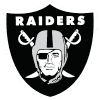Derek Carr
1.0% implied probability

+6500
65 to 1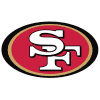Jimmy Garoppolo
1.0% implied probability

+8000
80 to 1Alvin Kamara
0.8% implied probability

+8000
80 to 1Ben Roethlisberger
0.8% implied probability

+10000
100 to 1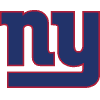Saquon Barkley
0.6% implied probability

+10000
100 to 1Taylor Heinicke
0.6% implied probability

No changes have been recorded yet.
+10000
100 to 1Nick Chubb
0.6% implied probability

+10000
100 to 1Daniel Jones
0.6% implied probability

+10000
100 to 1Trevor Lawrence
0.6% implied probability

+10000
100 to 1Amari Cooper
0.6% implied probability

No changes have been recorded yet.
+10000
100 to 1Taysom Hill
0.6% implied probability

+10000
100 to 1Aaron Donald
0.6% implied probability

No changes have been recorded yet.
+10000
100 to 1Antonio Brown
0.6% implied probability

No changes have been recorded yet.
+10000
100 to 1Drew Lock
0.6% implied probability

+10000
100 to 1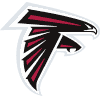Matt Ryan
0.6% implied probability

+10000
100 to 1Tyrod Taylor
0.6% implied probability

+10000
100 to 1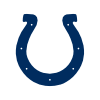Jonathan Taylor
0.6% implied probability

+10000
100 to 1Mac Jones
0.6% implied probability

+10000
100 to 1Raheem Mostert
0.6% implied probability

No changes have been recorded yet.
+10000
100 to 1Jacoby Brissett
0.6% implied probability

No changes have been recorded yet.
+10000
100 to 1Ezekiel Elliott
0.6% implied probability

+10000
100 to 1DeAndre Hopkins
0.6% implied probability

+10000
100 to 1Ryan Tannehill
0.6% implied probability

+10000
100 to 1Trey Lance
0.6% implied probability

+10000
100 to 1Antonio Gibson
0.6% implied probability

No changes have been recorded yet.
+10000
100 to 1Dalvin Cook
0.6% implied probability

+10000
100 to 1CeeDee Lamb
0.6% implied probability

No changes have been recorded yet.
+10000
100 to 1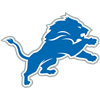D'Andre Swift
0.6% implied probability

No changes have been recorded yet.
+10000
100 to 1Davante Adams
0.6% implied probability

+10000
100 to 1Carson Wentz
0.6% implied probability

+10000
100 to 1Cooper Kupp
0.6% implied probability

+10000
100 to 1Jared Goff
0.6% implied probability

+10000
100 to 1Deebo Samuel
0.6% implied probability

No changes have been recorded yet.
+15000
150 to 1Will Fuller
0.4% implied probability

No changes have been recorded yet.
+15000
150 to 1Terry McLaurin
0.4% implied probability

No changes have been recorded yet.
+15000
150 to 1Tyler Lockett
0.4% implied probability

No changes have been recorded yet.
+15000
150 to 1Courtland Sutton
0.4% implied probability

No changes have been recorded yet.
+15000
150 to 1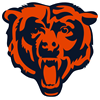Tarik Cohen
0.4% implied probability

No changes have been recorded yet.
+15000
150 to 1Kenyan Drake
0.4% implied probability

No changes have been recorded yet.
+15000
150 to 1James Robinson
0.4% implied probability

No changes have been recorded yet.
+15000
150 to 1Julio Jones
0.4% implied probability

+15000
150 to 1Clyde Edwards-Helaire
0.4% implied probability

+15000
150 to 1Mike Evans
0.4% implied probability

+15000
150 to 1Allen Robinson
0.4% implied probability

+15000
150 to 1Tyreek Hill
0.4% implied probability

+15000
150 to 1Calvin Ridley
0.4% implied probability

+15000
150 to 1George Kittle
0.4% implied probability

+15000
150 to 1Justin Jefferson
0.4% implied probability

+20000
200 to 1Chris Godwin
0.3% implied probability

No changes have been recorded yet.
+20000
200 to 1Odell Beckham
0.3% implied probability

+20000
200 to 1Melvin Gordon
0.3% implied probability

+20000
200 to 1Myles Gaskin
0.3% implied probability

No changes have been recorded yet.
+20000
200 to 1Stefon Diggs
0.3% implied probability

+20000
200 to 1Chris Carson
0.3% implied probability

No changes have been recorded yet.
+20000
200 to 1Leonard Fournette
0.3% implied probability

+20000
200 to 1Kareem Hunt
0.3% implied probability

No changes have been recorded yet.
+20000
200 to 1Aaron Jones
0.3% implied probability

+20000
200 to 1Mitchell Trubisky
0.3% implied probability

No changes have been recorded yet.
+20000
200 to 1Joe Mixon
0.3% implied probability

+20000
200 to 1Austin Ekeler
0.3% implied probability

+20000
200 to 1Miles Sanders
0.3% implied probability

+20000
200 to 1Todd Gurley
0.3% implied probability

No changes have been recorded yet.
+20000
200 to 1David Montgomery
0.3% implied probability

+20000
200 to 1Josh Jacobs
0.3% implied probability

+20000
200 to 1Adam Thielen
0.3% implied probability

No changes have been recorded yet.
+20000
200 to 1Le'Veon Bell
0.3% implied probability

No changes have been recorded yet.
+20000
200 to 1Keenan Allen
0.3% implied probability

+20000
200 to 1Ryan Fitzpatrick
0.3% implied probability

+20000
200 to 1Justin Fields
0.3% implied probability

+20000
200 to 1Travis Kelce
0.3% implied probability